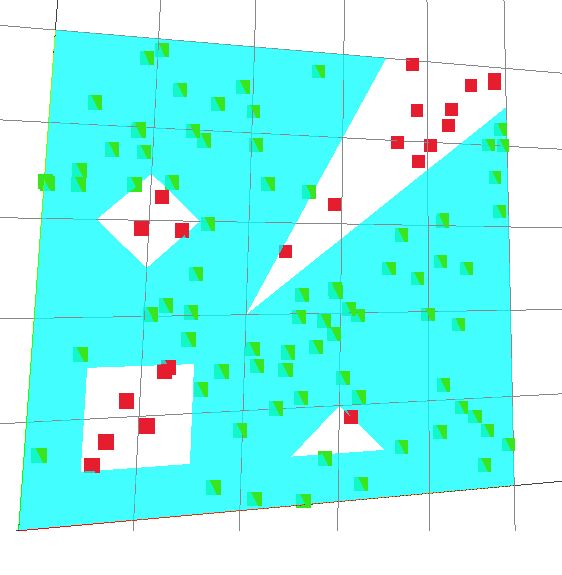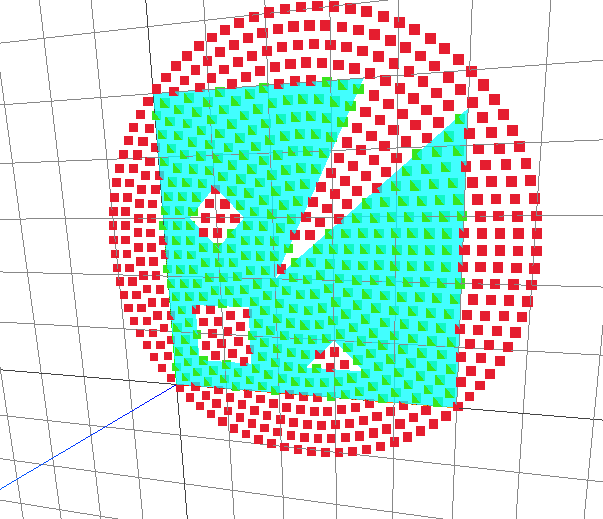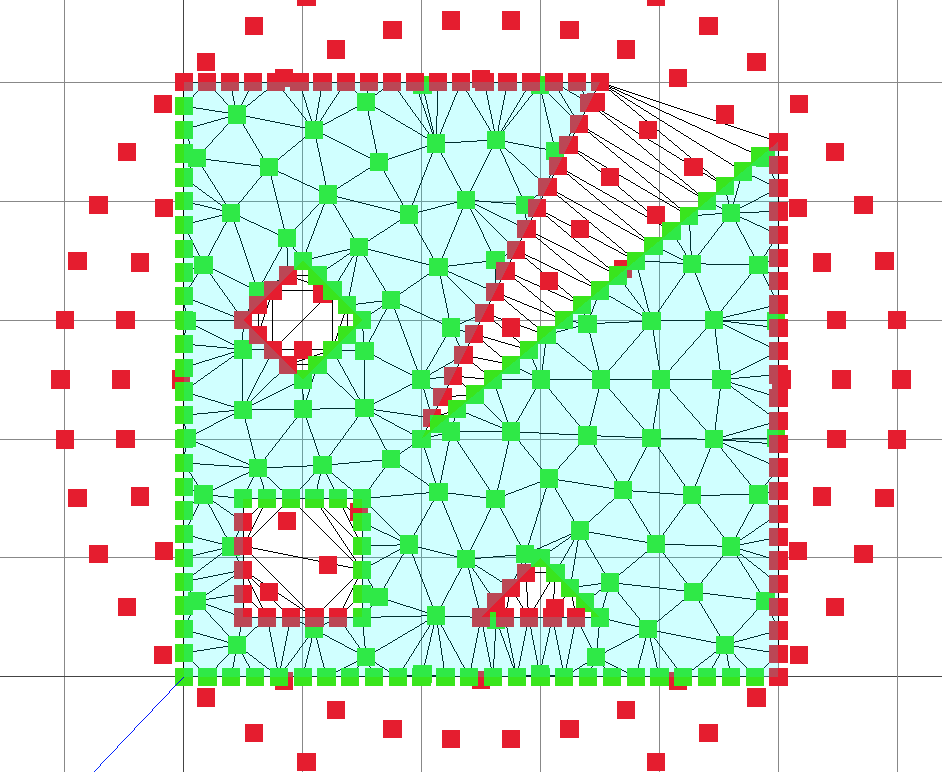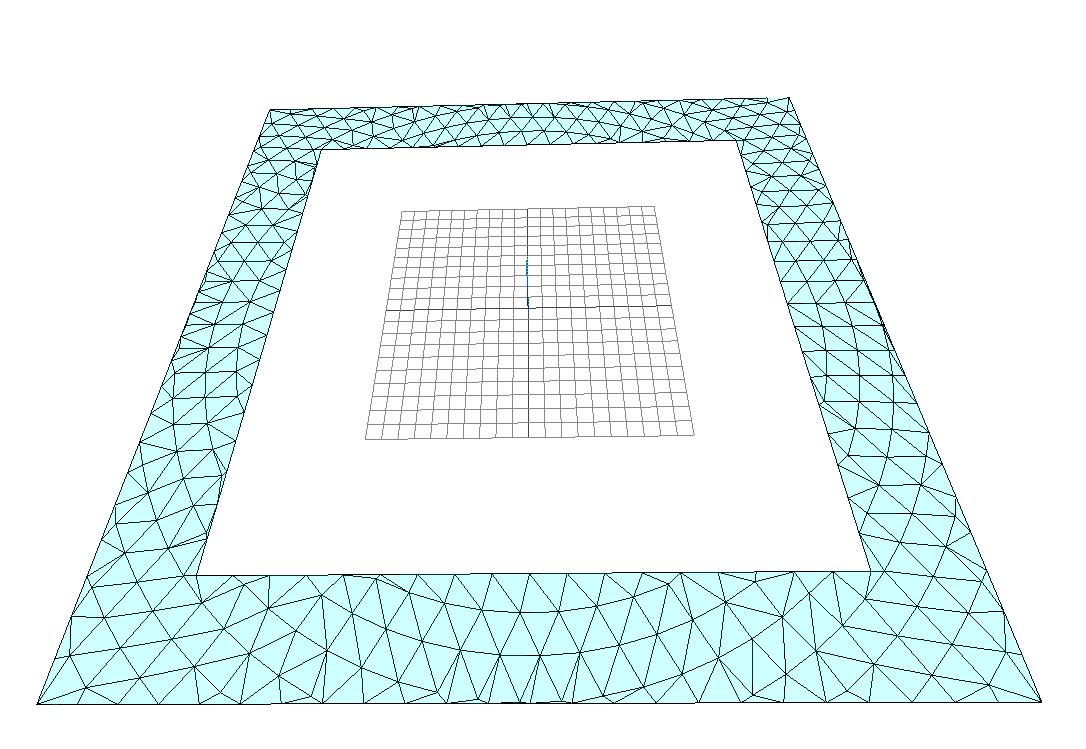Now that the user interface for the concrete tool is up and running, I need to start working on the concrete analysis.

The first order of business is a meshing algorithm for the concrete shape.I do not know exactly which direction this meshing algorithm is going to head, but every direction I look, it appears as though I will need some code to check if a given point falls within an enclosed polygon. The pretty pictures above show the code in action, if the point falls within the polygon, it is painted green, if not, red.

After some research, it appeared as though the ray caster algorithm would be the ticket to solve this problem.

The code to check if the point falls within any given abritary polygon is shown below:

```function ray_casting(point, testPoly, holePolys) {
var n = testPoly.length;
var count = 0
var holeCount = 0
var x = point;
var y = point;

for(var i=0; i <n; ++i) {
if (i == n-1) {
var side = {
a: {
x: testPoly[i],
y: testPoly[i]
},
b: {
x: testPoly,
y: testPoly
}
}
var x1 = side.a.x
var x2 = side.b.x
var y1 = side.a.y
var y2 = side.b.y
if (y < y1 != y < y2 && x < (x2-x1)*(y-y1)/ (y2-y1)+x1) {
count +=1
}
}
else {
var side = {
a: {
x: testPoly[i],
y: testPoly[i]
},
b: {
x: testPoly[i+1],
y: testPoly[i+1]
}
}
var x1 = side.a.x
var x2 = side.b.x
var y1 = side.a.y
var y2 = side.b.y
if (y < y1 != y < y2 && x < (x2-x1)*(y-y1)/ (y2-y1)+x1) {
count +=1
}
}
}
for (var holePoly of holePolys) {
var nHole = holePoly.length;
for(var i=0; i <nHole; ++i) {
if (i ==nHole-1){
var side = {
a: {
x: holePoly[i],
y: holePoly[i]
},
b: {
x: holePoly,
y: holePoly
}
}
var x1 = side.a.x
var x2 = side.b.x
var y1 = side.a.y
var y2 = side.b.y
if (y < y1 != y < y2 && x < (x2-x1)*(y-y1)/ (y2-y1)+x1) {
holeCount +=1
}
}
else{
var side = {
a: {
x: holePoly[i],
y: holePoly[i]
},
b: {
x: holePoly[i+1],
y: holePoly[i+1]
}
}
var x1 = side.a.x
var x2 = side.b.x
var y1 = side.a.y
var y2 = side.b.y
if (y < y1 != y < y2 && x < (x2-x1)*(y-y1)/ (y2-y1)+x1) {
holeCount +=1
}
}
}
}
if (count % 2 == 0 || count == 0 ) {
return [false, count, holeCount]
}
else if (holeCount % 2 == 1) {
return [false, count, holeCount]
}
else {
return [true, count, holeCount]
}
}```

The algorithm is very simple, given a point, cast an inifinite line to the right. If the line crosses the polygon an even number of times, the point is outside the polygon.

Adding in some more code to test for holes and we are able to quickly tell if the point is inside or outisde the polygon with holes.The code can be found on github.

A tease for the future: